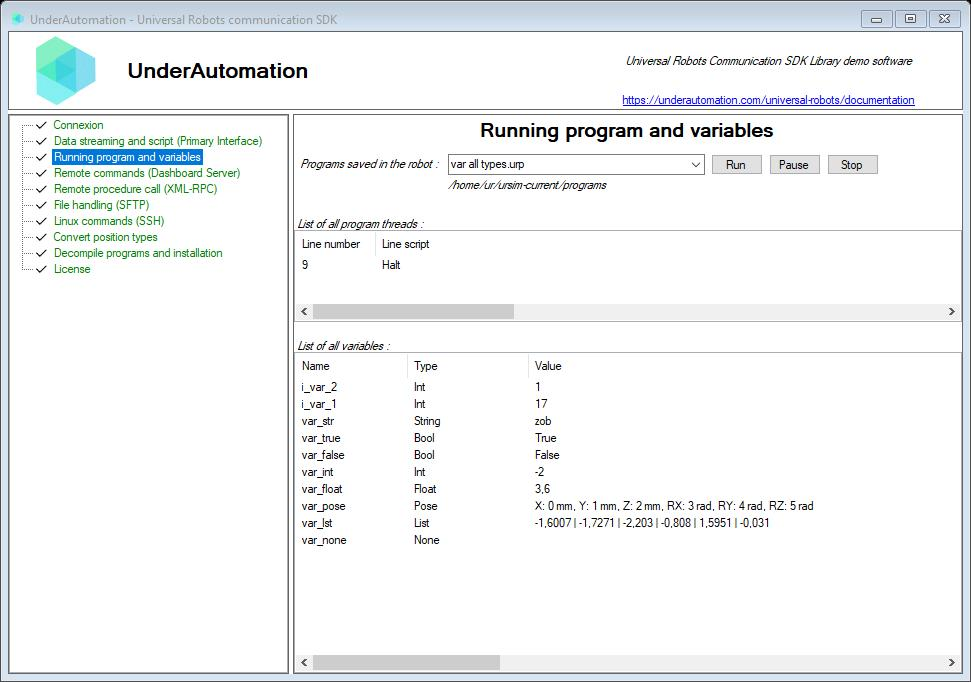In this chapter, we call "variable" a global variable to the UR controller, it can be a program variable or an installation variable.

In the library, whatever the protocol used to manipulate variables, a variable is represented by the `GlobalVariable` class which contains the name and value of the variable. This class inherits from `GlobalVariableValue` which contains only the value.

The Dashboard server protocol allows the robot to be asked for the value of a variable.

The function that implements this command is `GetVariable`. A GlobalVariableValue object is returned which contains the name and value of the variable. The type is estimated from the value.

```// Read variable called "myVar"var request = robot.Dashboard.GetVariable("myVar");
if (request.Succeed){    GlobalVariable variable = request.Value;    // variable.Name    // variable.Type    // variable.Value    // variable.ToMatrix();    // variable.ToFloat();    // variable.To...();}```

## Read variables with Primary Interface

Primary Interface raises an event when the list of variables or the value of a variable changes. The library maintains the last received value of each variable. This means you can access the name, type and value of variables at any time.

Warning: if your application connects while a program is running, you will only be notified of the list of variables when there is a change. It is therefore possible that the list is empty because Primary Interface only reacts to changes.

Note: the library adapts to the controller's firmware version. Indeed, from version 3.2, then 5.9 the way to decode the variables is different, but it is seemless for the developer.

2 events allow you to be notified when the list of variables changes (`ListUpdated`) or when one of the variables changes value (`ValuesUpdated`).

Primary interface has a `GlobalVariables` property that allows access to the list of variables and associated events.

```// Be notified when the list of variables changesrobot.PrimaryInterface.GlobalVariables.ListUpdated += GlobalVariables_ListUpdated;
// Be notified when at least one variable changes valuerobot.PrimaryInterface.GlobalVariables.ValuesUpdated += GlobalVariables_ValuesUpdated;
// Get the list of all variablesGlobalVariable[] variables = robot.PrimaryInterface.GlobalVariables.GetAll();
if (variables.Length == 0) return;
// Get first variablevar variable = variables;
// Get variable namestring name = variable.Name;
// Get variable generic valueobject value = variable.Value;
// Get its value according to its typeswitch (variable.Type){    case GlobalVariableTypes.None:        // Variable is empty or doesn't exist        break;    case GlobalVariableTypes.String:        string stringValue = variable.ToString();        break;    case GlobalVariableTypes.List:        GlobalVariableValue[] listValue = variable.ToList();        break;    case GlobalVariableTypes.Pose:        Pose poseValue = variable.ToPose();        break;    case GlobalVariableTypes.Bool:        bool boolValue = variable.ToBool();        break;    case GlobalVariableTypes.Int:        int intValue = variable.ToInt();        break;    case GlobalVariableTypes.Float:        float floatValue = variable.ToFloat();        break;    case GlobalVariableTypes.Matrix:        System.Array matrixValue = variable.ToMatrix();        break;}```

## Try it with the Windows example## Classes

Members of Common.GlobalVariable :
`public class GlobalVariable : GlobalVariableValue {     // Variable name    public string Name { get; }     // Last time the variable was sampled    public TimeSpan Time { get; }}`
Members of Common.GlobalVariableValue :
`public class GlobalVariableValue {     // Estimate variable value from its string representation    public static GlobalVariableValue Parse(string message)     // Returns variable value if type is Bool. Il type is Float or Int, it returns True if value is not 0. Else, it returns false    public bool ToBool()     // Returns variable value if type is Float. Il type is int, it casts it to float. If Type is bool, it returns 1 or 0. Else it returns NaN    public float ToFloat()     // Returns variable value if type is Int. Il type is Float, it tries to cast it to int. If Type is bool, it returns 1 or 0. Else it returns 0    public int ToInt()     // Returns an array of GlobalVariableValue if Type is List. Else, null is returned    public GlobalVariableValue[] ToList()     // Return variable value GlobalVariable[,] if variable is a matrix. First dimension is row index and second dimension is column index.    // Use GetLength(0) to get row number and GetLength(1) to get column count    public Array ToMatrix()     // Returns a Pose if Type is Pose. Else, null is returned    public Pose ToPose()     // Returns a string that describes the variable, regardless of its type    public override string ToString()     // Type of a variable    public GlobalVariableTypes Type { get; }     // Value of the variable    public object Value { get; }}`
Members of Common.GlobalVariableTypes :
`public enum GlobalVariableTypes {     // Variable value is bool    Bool = 4     // Variable value is float    Float = 6     // Variable value is int    Int = 5     // Variable value is an array : GlobalVariableValue[]    List = 2     // Variable value is a matrix    Matrix = 7     // Variable value is null, the value has not been assigned yet    None = 0     // Variable value is a UnderAutomation.UniversalRobots.Pose    Pose = 3     // Variable value is a System.String    String = 1}`
Members of PrimaryInterface.GlobalVariables :
`public class GlobalVariables {     // Indicates which decoder is used used to read variables according to firmware version    public GlobalVariablesFirmwareVersion FirmwareVersion { get; }     // Returns a list of all variables declared in the robot    public GlobalVariable[] GetAll()     // Get a variable by its name. Null is returned if the variable doesn&apos;t exist    public GlobalVariable GetByName(string name)     // Event raised whan the variable list changed. For example, after a program starts    public event EventHandler<GlobalVariablesEventArgs> ListUpdated     // Return a string where each line contains type, name and variable value    public override string ToString()     // Event raised at 10Hz when variable values are updated    public event EventHandler<GlobalVariablesEventArgs> ValuesUpdated}`